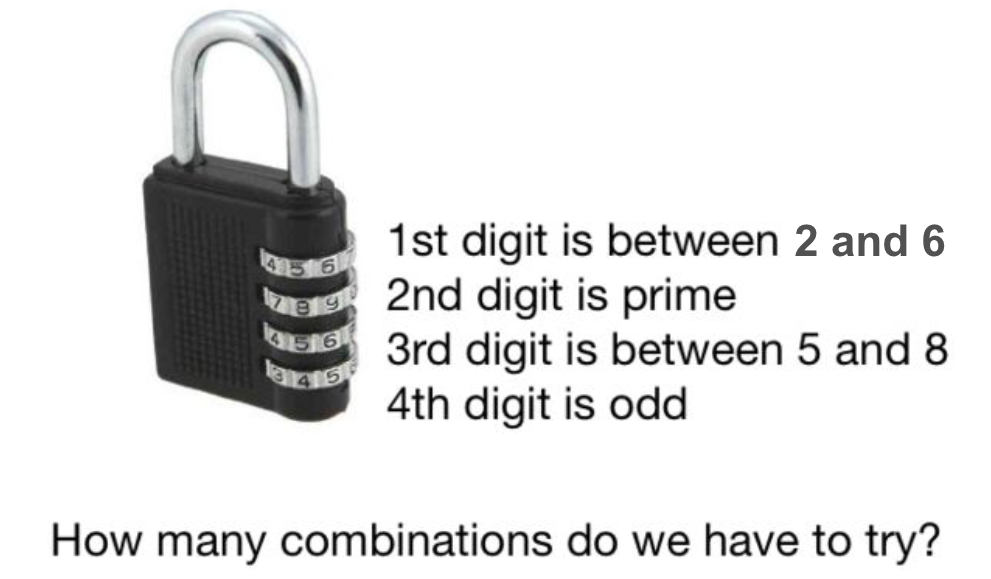# Breaking Into Luggage

Probability Level 2After a few hours, David finally arrived at his hotel. When he wanted to open his briefcase; he couldn't remember the 4-digit combination code. He was, however, able to recall:

• The $1^\text{st}$ digit is a number between 2 and 6 inclusive.
• The $2^\text{nd}$ digit is a prime number between 2 and 9 inclusive.
• The $3^\text{rd}$ digit is a number between 5 and 8 inclusive.
• The $4^\text{th}$ digit is an odd number.

What is the total number of possible combination code to the briefcase?

×